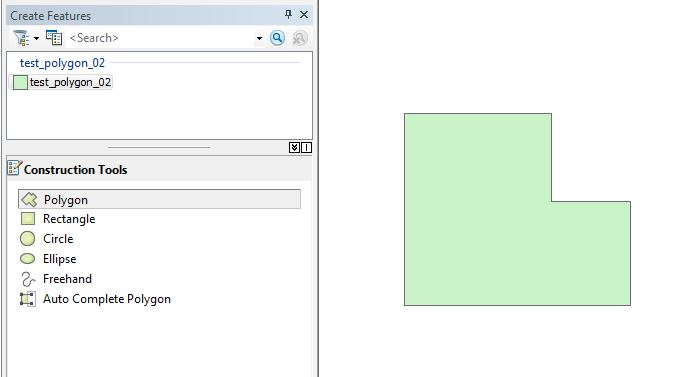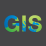# Custom polygon shapes for drawing ?

5394
17
12-11-2014 02:14 PMNew Contributor

So I would like to create additional options for custom shapes when editing a polygon feature. Something to the same functionality of the 'Construction Tools' as pictured below, but with a greater variety of shapes (like a triangle, pentagon, irregular shape as below, etc).Wondering what the best method would be to go about this? I'm assuming it's not possible to add custom shapes directly into the already existing Construction Tools panel (tho I'm not technically versed enough to know).. but in that case would writing an Add-In be the best way? Is it possible?

..I probably won't be doing this by myself unless it requires minimal coding experience, but I'd really appreciate if someone can point me to the correct basic approach!

17 RepliesOccasional Contributor II

Hello Kira,

It is possible to create custom shapes. It does, however, require python code to perform it. I can pass some code along to you. It basically works, in a manner, where the user drops a point and then draws a polygon. I used it to create many polygons based on zoning. The polygons were basic shapes based on length and height. If you had several custom shapes, then code would have to account for the dimensions.Also, you would have to start an edit session to orient the shapefiles.

Let me know if you want the code

JamesNew Contributor

Yes, I'd love to see that code!That'd be brilliant. Thanks for the response~Occasional Contributor II

I would love to see the code as well if that would be possible. This could same me quite a bit of time. Thanks!Occasional Contributor II
```import arcpy, os
arcpy.env.workspace = "U:\Tax\Special Projects\JEFitzgerald\CUVA\2014\CUVA_PYTHON_06252014_Final\CUVA.mdb"
pointList=[]
#Finds the field named File and deletes it
try:
with arcpy.da.UpdateCursor("GC_R20_Polygon",("File"),'[File] =\'Done\'') as cursor:
cntr = 1
for row in cursor:
cursor.deleteRow()
print "Record number "+ str(cntr) + " deleted"
cntr = cntr + 1
except Exception as e:
print e.message

#****  CODE for R20    ****#
try:
with arcpy.da.SearchCursor ("GC_R20", ["SHAPE@XY"]) as cursor:
for row in cursor:
xy = row
pointList.append(xy)

with arcpy.da.InsertCursor ("GC_R20_Polygon", ["SHAPE@"]) as U:
for pnt in pointList:
lowX = pnt
lowY = pnt
#   find the upper right
highX = lowX + 162.2539
highY = lowY + 123.2639
array = arcpy.Array([arcpy.Point(lowX, lowY),
arcpy.Point(lowX, highY),
arcpy.Point(highX, highY),
arcpy.Point(highX, lowY),
arcpy.Point(lowX, lowY)])
polygon = arcpy.Polygon(array)
U.insertRow([polygon])

#Populates a field with DONE so the delete cursor will find and delete.
#This will enable a clean attribute table so dublicates will be eliminated.
arcpy.CalculateField_management("GC_R20_Polygon","File", "\"Done\"", "PYTHON", "")
print "Process for new polygons is complete"
print "James E. Fitzgerald, GISP, GIS Specialist"
except:
print ```Occasional Contributor II

Hello,

With this code you have to create your shapefiles. I am new to Python Scripting so there may be excess to delete. Thanks,

JamesNew Contributor

James Fitzgerald, Thanks so much for posting this. I'm super new to Python, but I think I understand what the code is doing, but could you explain a little more how to implement it in ArcGIS? You mentioned to have an Edit session open.. Perhaps which parameters need to be changed or the defined 'workspace'? Not quite sure how that works. If I run this in Arc atm, in an open Edit session, I get "cannot open 'GC_R20_Polygon'".Occasional Contributor II

You have to create a point.shp (GC_R20). GC_R20.shp is what I used give me the starting point in terms of XY. Also, you have to have a polygon created too. I named it GC_R20_Polygon. I edited the original code to exclude unnecessary code.

```import arcpy, os
arcpy.env.workspace = "U:\Tax\Special Projects\JEFitzgerald\CUVA\2014\CUVA_PYTHON_06252014_Final\CUVA.mdb"
pointList=[]

try:
with arcpy. ["SHAPE@XY"]) as cursor:
for row in cursor:
xy = row
pointList.append(XY)

with arcpy.0_Polygon", ["SHAPE@"]) as U:
for pnt in pointList:
lowX = pnt
lowY = pnt
#   find the upper right
highX = lowX + 162.2539
highY = lowY + 123.2639
array = arcpy.Array([arcpy.Point
arcpy.Point(lowX, highY),
arcpy.Point(highX, highY),
arcpy.Point(highX, lowY),
arcpy.Point(lowX, lowY)])
polygon = arcpy.Polygon(array)
U.insertRow([polygon])

#Populates a field with DONE so the delete cursor will find and delete.
#This will enable a clean attribute table so dublicates will be eliminated.
arcpy.CalculateField_management("GC_R20_Polygon","File", "\"Done\"", "PYTHON", "")
print "Process for new polygons is complete"
except:
print   ```Occasional Contributor II

I think you accidentally removed parts that are necessary for the code to work? i.e Line 6 and Line 11.

On a side note, this code will work for 10.1 and up. the Data Access module was introduced at 10.1. I am only on 10and can't test it. But thanks anyways, I'll keep it in my back pocket.withNew Contributor

That gave me some syntax errors, but I edited the code a little (below) and created my own shape named GC_R20, with a polygon also named GC_R20.. I run it through the Python window in Arc and receive no errors, but nothing seems to happen either. ‌Should this add a polygon to a template somewhere, or should it work if I start drawing in the Edit session?

(for some reason it's not letting me apply the code style atm..)

 import arcpy, os    arcpy.env.workspace = "D:/TESTING"   pointList=[]      try:           with arcpy.da.SearchCursor ("GC_R20", ["SHAPE@XY"]) as cursor:          for row in cursor:                xy = row                pointList.append(XY)                   with arcpy.da.InsertCursor ("GC_R20", ["SHAPE@"]) as U:             for pnt in pointList:                lowX = pnt                lowY = pnt            #   find the upper right                highX = lowX + 162.2539                highY = lowY + 123.2639                array = arcpy.Array([arcpy.Point(lowX, lowY),                               arcpy.Point(lowX, highY),                                 arcpy.Point(highX, highY),                                 arcpy.Point(highX, lowY),                                 arcpy.Point(lowX, lowY)])                polygon = arcpy.Polygon(array)                U.insertRow([polygon])                        #Populates a field with DONE so the delete cursor will find and delete.        #This will enable a clean attribute table so dublicates will be eliminated.        arcpy.CalculateField_management("GC_R20","File", "\"Done\"", "PYTHON", "")        print "Process for new polygons is complete"     except:        print